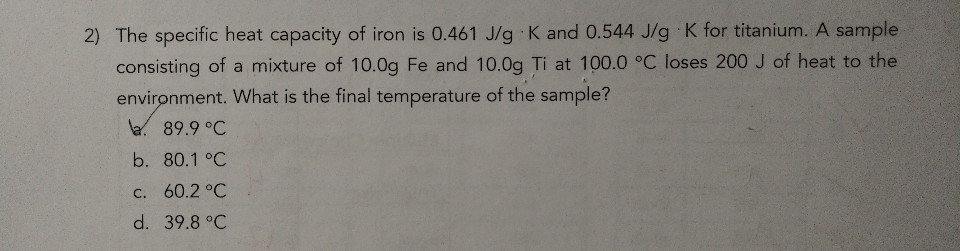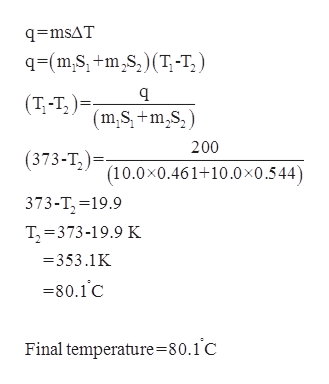# 2) The specific heat capacity of iron is 0.461 J/g K and 0.544 J/g K for titanium. A sampleconsisting of a mixture of 10.0g Fe and 10.0g Ti at 100.0 °C loses 200 J of heat to theenvironment. What is the final temperature of the sample?a. 89.9 °Cb. 80.1 °Cc. 60.2 °Cd. 39.8 °C

Question
224 viewshelp_outlineImage Transcriptionclose2) The specific heat capacity of iron is 0.461 J/g K and 0.544 J/g K for titanium. A sample consisting of a mixture of 10.0g Fe and 10.0g Ti at 100.0 °C loses 200 J of heat to the environment. What is the final temperature of the sample? a. 89.9 °C b. 80.1 °C c. 60.2 °C d. 39.8 °C fullscreen
check_circle

Step 1

Given,

Specific heat capacity of iron = 0.461J/gK.

Specific heat capacity of titanium = 0.544J/gK.

Mass of iron = 10.0g.

Mass of titanium = 10.0g.

Heat lost = 200J.

Temperature = 100.00C = 373K.

Step 2

Final temperature of ...help_outlineImage Transcriptioncloseq=msAT q=(m,S, +m,s,)(T,-T, ) (Т-т,)- (m,S, +m,S,) 200 (373-T,)= (10.0x0.461+10.0×0.544) 373-T, =19.9 T, =373-19.9 K = 353.1K =80.1c Final temperature=80.1°C fullscreen

### Want to see the full answer?

See Solution

#### Want to see this answer and more?

Solutions are written by subject experts who are available 24/7. Questions are typically answered within 1 hour.*

See Solution
*Response times may vary by subject and question.
Tagged in

### Physical Chemistry